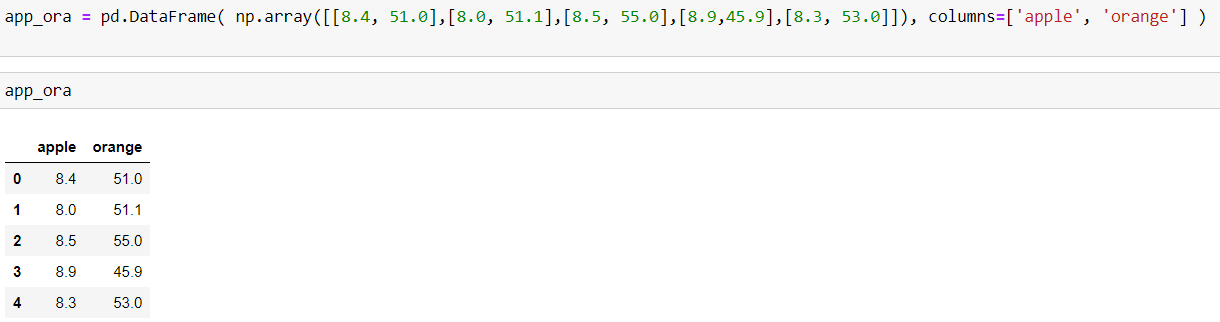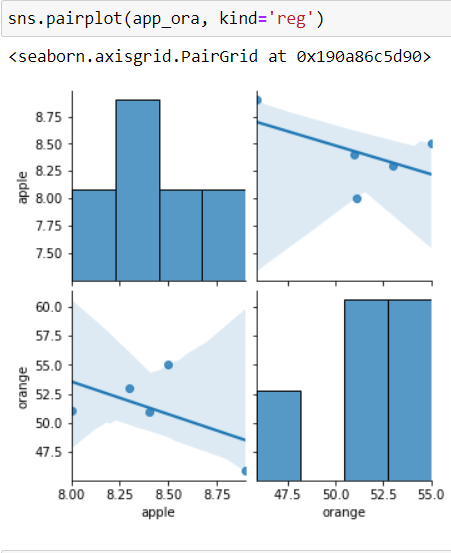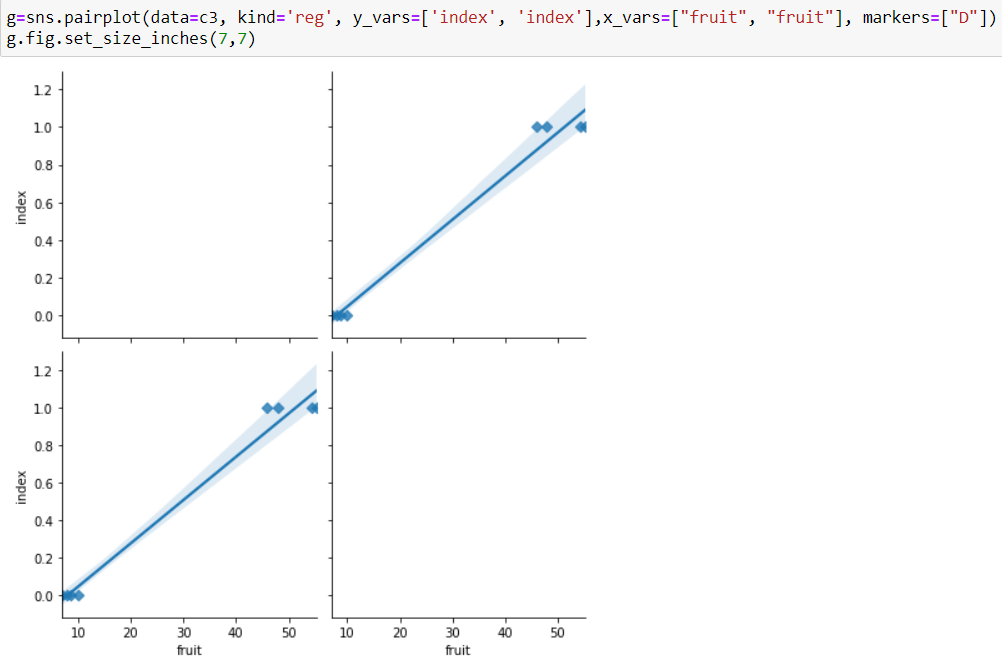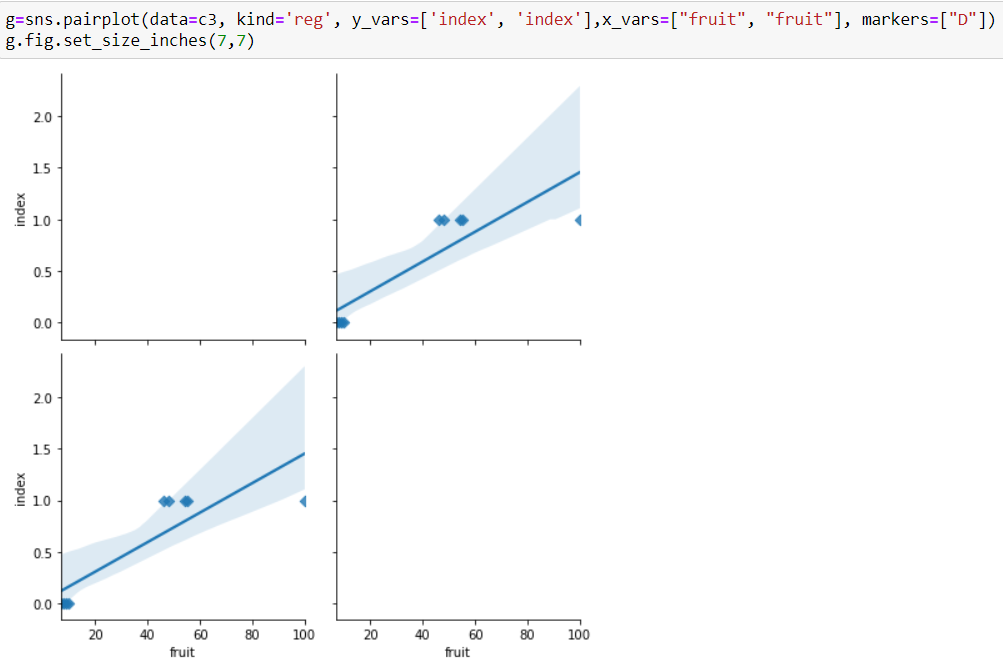# A different class of Machine LearningPandas DataFrame with apple and orange vit-c mglinear reg plot of classification problema linear regression type plot for a categorical problem.Outliers make linear regression less predictive and useful.